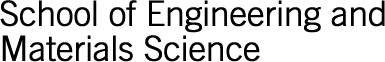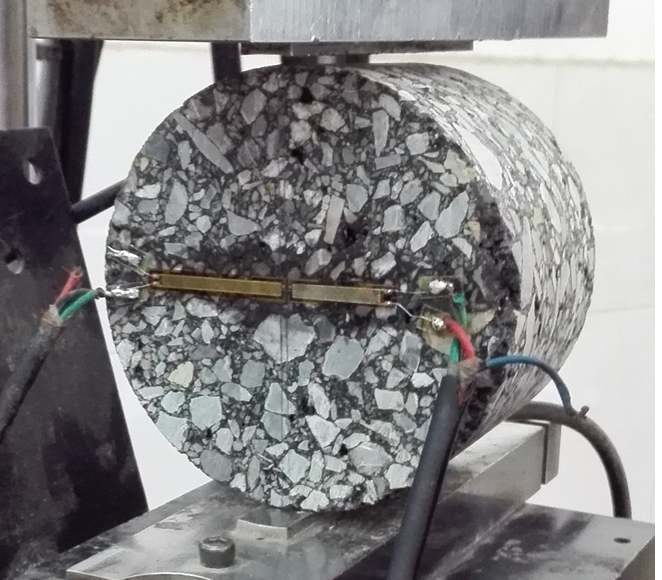CloseOne of the difficulties in dealing with bimodular materials including composites, rock and asphalt-mixture material is the discontinuity of Young's modulus when the principal stress changes the sign, i.e. from the tensile state to compressive stress state. According to the general elastic theory proposed by Ambartsumyan, there are two kinds of domains in which the coefficients of elasticity are constants. This discontinuity of Young's modulus causes divergence in the computational procedure. Two continuous modes for bimodular materials are investigated in this project. The nonlinear equilibrium equations have been formulated with a continuous constitute equation of stress and strain. The finite element and meshless finite block methods will be developed in solving the nonlinear problems for bimodular materials.